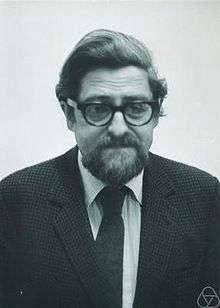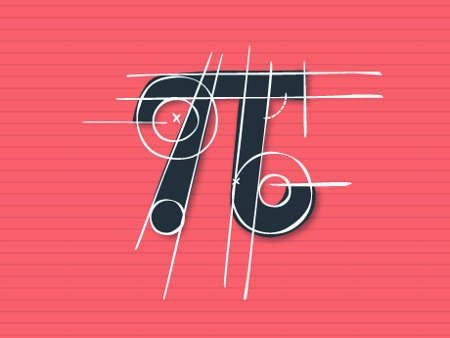### Louis Nirenberg, the geometry and the Abel Prize

http://t.co/rTEYZLbVDZ by @ulaulaman about #AbelPrize #LouisNirenbergGreat news: John Nash and Louis Nirenberg win the Abel Prize for 2015:
The Norwegian Academy of Sciences and Letters has decided to award the Abel Prize for 2015 to the American mathematicians John F. Nash Jr. and Louis Nirenberg “for striking and seminal contributions to the theory of nonlinear partial differential equations and its applications to geometric analysis.” The President of the Academy, Kirsti Strøm Bull, announced the new laureates today 25 March. They will receive the Abel Prize from His Majesty King Harald at a ceremony in Oslo on 19 May.
While Nash is known for his contribution to game theory with Nash equilibria, Nirenberg is
considered one of the outstanding analysts of the twentieth century. He has made fundamental contributions to linear and nonlinear partial differential equations and their application to complex analysis and geometry.
His first result was the completion of the solution of a problem in differential geometry, starting from the 1916 work of Weyl (read On the work of Louis Nirenberg by Simon Donaldson, pdf).
The statement is very simple: an abstract Riemannian metric on the 2-sphere with positive curvature can be realised by an isometric embedding in $R^3$.
In 1994 he received the Steele Prize by the American Mathematical Society. In that occasion, the Society writes (via MacTutor) a good summary of his activity:
Nirenberg is a master of the art and science of obtaining and applying a priori estimates in all fields of analysis. A minor such gem is the useful set of Garliardo-Nirenberg inequalities. A high point is his joint research with [Shmuel] Agmon and [Avron] Douglis on a priori estimates for general linear elliptic systems, one of the most widely quoted results in analysis. Another is his fundamental paper with Fritz John on functions of bounded mean oscillation which was crucial for the later work of [Charles] Fefferman on this function space. Nirenberg has been the centre of many major developments. His theorem with his student, Newlander, on almost complex structures has become a classic. In a paper building on earlier estimates of [Alberto] Calderón and [Antoni] Zygmund, he and [Joseph] Kohn introduced the notion of a pseudo-differential operator which helped to generate an enormous amount of later work. His research with [François] Trèves was an important contribution to the solvability of general linear PDEs. Some other highlights are his research on the regularity of free boundary problems with [David] Kinderlehrer and [Joel] Spuck, existence of smooth solutions of equations of Monge-Ampère type with [Luis] Caffarelli and Spuck, and singular sets for the Navier-Stokes equations with Caffarelli and [Robert] Kohn. His study of symmetric solutions of non-linear elliptic equations using moving plane methods with [Basilis] Gidas and [Wei Ming] Ni and later with [Henri] Berestycki, is an ingenious application of the maximum principle.
I hope that you can appreciate the list of collaboration performed by Nirenberg: indeed he is one of the most collaborative mathematician in the word. In particular for this reason I'm really happy for the award to Nirenberg.
Read also: Interview with Louis Nirenberg (pdf)

### Pi day: Zilienski, Wallis and the square

http://t.co/SopVLuLJHM by @ulaulaman #piday
A good pi day to all readers! I hope that the following post, that I cannot review after the first writing, could be interesting to all!The technique used by the ancient Greek for their geometric constructions was called "ruler and compass". In this way you can build a lot of regular polygons, for example, but there are three problems that are impossible unless you use different techniques: the angle trisection, doubling the cube, squaring the circle.
In particular for the squaring, it is easy to calculate the relation between the radius $r$ of the circke and the side $l$ of the square with the same area of the starting circle: $L = \sqrt {\pi} r$ Now, since $\pi$ is a transcendental number, the formula above is the simplest representation of the impossibility of squaring the circle using ruler and compass: with these devices you can treat rational and irrational numbers, such as $\sqrt{2}$ (in this case simply draw a square of side 1).
So, using these two tools it is possible to obtain an approximate construction and, therefore, a corresponding approximate value for $\pi$: during the XX century there are produced a lot of approximations, for example by CD Olds (1963), Martin Gardner (1966), Benjamin Bold (1982). They are all variations of the geometric construction discovered by Srinivasa Ramanujan in 1913, that approached $\pi$ with the fraction $\frac{355}{113} = 3.1415929203539823008 \dots$ right up to the sixth decimal place.
In 1914, Ramanujan discovered a more accurate approximation (to eight decimal places), always using ruler and compass: $\left (9^2 + \frac{19}{22}^2 \right)^{1/4} = \sqrt{\frac{2143}{22}} = 3.1415926525826461252 \dots$

### Riemann zeta function's fractal

#arXiv #abstract on #zetafunction and #fractalsvia imgur
The quadratic Mandelbrot set has been referred to as the most complex and beautiful object in mathematics and the Riemann Zeta function takes the prize for the most complicated and enigmatic function. Here we elucidate the spectrum of Mandelbrot and Julia sets of Zeta, to unearth the geography of its chaotic and fractal diversities, combining these two extremes into one intrepid journey into the deepest abyss of complex function space.
Fractal Geography of the Riemann Zeta Function by Chris King